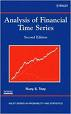Analysis of Financial Time Series by Ruey Tsay

A good introduction to the analysis of time series data, Tsay covers univariate and multivariate autoregressive models well (ARCH, GARCH, ARMA, VARMA), neural networks, Ito process, and Extreme Value models are intro'd. Tsay includes review questions at the end of each chapter that use several excel and S-Plus packages. Given the state of R, the exercises could and should be done with R instead.

Recent studies of stock returns tend to use scale mixture or finite mixture of normal distributions. Looks like Mandelbrot's ideas have had an influence ( Finance studies using fatter tail distributions than normal ).
In the finance literature, it is common to assume that an asset return series is weakly stationary. This assumption can be checked empirically provided that a sufficient number of historical returns are available. For example, one can divide the data into subsamples and check the consistency of results obtained.